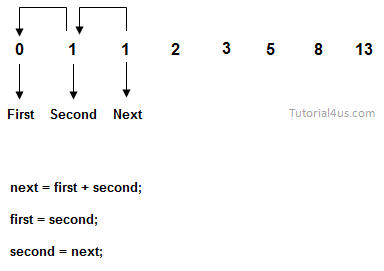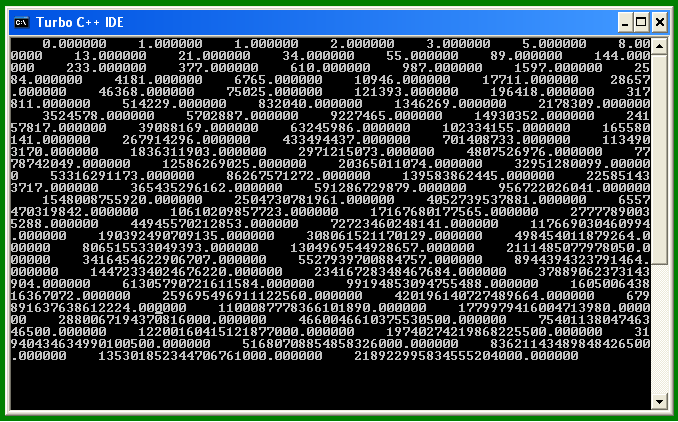# Print Fibonacci Series in C

## Fibonacci Series Program in C

Fibonacci series is in the form of 0, 1, 1, 2, 3, 5, 8, 13, 21,...... To find Fibonacci series you need to add two previous terms/digits and get next term/number.## Print fibonacci series

```#include<stdio.h>
#include<conio.h>

void main()
{
int i,no, first=0, second=1, next;
clrscr();
first=0;
second=1;
printf("Enter nubmer of terms for Series: ");
scanf("%d",&no);
printf("Fibonacci series are:\n");
for(i=0; i<no; i++)
{
printf("%d\n",first);
next = first + second;
first = second;
second = next;
}
getch();
}
```

## Output

```Enter nubmer of terms for Series: 7
Fibonacci series are:
0
1
1
2
3
5
8
```

## program fibonacci series upto 100

```#include<stdio.h>
#include<conio.h>

void main()
{
double i, first, second, next;
clrscr();
first=0;
second=1;
for(i=1; i<=100; i++)
{
printf(" %lf",first);
next=first+second;
first=second;
second=next;
}
getch();
}
```

OutputWIFI CCTV Camera